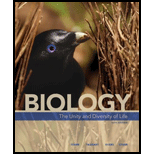Chapter 3, Problem 3SQBiology: The Unity and Diversity o...

15th Edition
Cecie Starr + 3 others
ISBN: 9781337408332

Solutions

Chapter
SectionBiology: The Unity and Diversity o...

15th Edition
Cecie Starr + 3 others
ISBN: 9781337408332
Textbook Problem

_______ groups are the “acid” part of amino acids and fatty acids. a. Hydroxyl (—OH) b. Carboxyl (—COOH) c. Methyl (—CH3) d. Phosphate (—PO4)

Summary Introduction

Concept Introduction: Amino acids and fatty acids are organic acids that are majorly composed of carbon and hydrogen atoms. Amino acids are the basic unit of proteins. Fatty acids are the basic unit of lipids. The acidic nature of these acids is given by a specific functional group called as carboxyl group.

Explanation

Reasons for correct statement:

The carboxyl functional group has the molecular formula CHO2. The general formula of a carboxylic acid is R–COOH, where R is any alkyl or aromatic group to which the carboxyl group can bind to. Carboxyl group is seen in amino acids and fatty acids, which makes them as organic acids.

Option b. is given as “Carboxyl (—COOH)”

As carboxyl group is present in amino acid and fatty acids giving them a pH less than 7, thus making them acidic, option b. is correct.

Reasons for the incorrect statements:

Option a. is given as “Hydroxyl (—OH)”.

Hydroxyl group is part of carboxyl group. Hydroxyl group alone will impart alkaline nature to compounds...

Still sussing out bartleby?

Check out a sample textbook solution.

See a sample solution

The Solution to Your Study Problems

Bartleby provides explanations to thousands of textbook problems written by our experts, many with advanced degrees!

Get Started